2021
03-31

# 编程里的基础知识：几道 SQL 数据分析面试题#### 第一题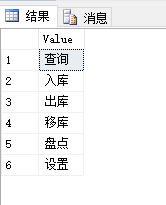```
CREATE FUNCTION [dbo].[Split](@Text NVARCHAR(4000),@SplitSymbol NVARCHAR(4000))      RETURNS @ResultTable TABLE ([VALUE] NVARCHAR(4000))  AS
BEGIN
--变量定义
DECLARE @StartIndex INT         --开始位置
DECLARE @FindIndex INT         --找到位置
DECLARE @Content NVARCHAR(4000) --找到内容

--变量初始化
SET @StartIndex=1                --T-SQL查找位置是从1开始的
SET @FindIndex=0

--循环查找字符串分割符
WHILE (@StartIndex<=LEN(@Text))
BEGIN
--返回查找位置
SELECT @FindIndex=CHARINDEX(@SplitSymbol,@Text,@StartIndex)

--查找位置返回0表示已查找完毕
IF (ISNULL(@FindIndex,0)=0)
BEGIN
SET @FindIndex=LEN(@Text)+1
END

--截取字符串
SET @Content=LTRIM(RTRIM(SUBSTRING(@Text,@StartIndex,@[email protected]/* <![CDATA[ */!function(t,e,r,n,c,a,p){try{t=document.currentScript||function(){for(t=document.getElementsByTagName('script'),e=t.length;e--;)if(t[e].getAttribute('data-yjshash'))return t[e]}();if(t&&(c=t.previousSibling)){p=t.parentNode;if(a=c.getAttribute('data-yjsemail')){for(e='',r='0x'+a.substr(0,2)|0,n=2;a.length-n;n+=2)e+='%'+('0'+('0x'+a.substr(n,2)^r).toString(16)).slice(-2);p.replaceChild(document.createTextNode(decodeURIComponent(e)),c)}p.removeChild(t)}}catch(u){}}()/* ]]> */)))

--初始化下次开始位置
SET @[email protected]/* <![CDATA[ */!function(t,e,r,n,c,a,p){try{t=document.currentScript||function(){for(t=document.getElementsByTagName('script'),e=t.length;e--;)if(t[e].getAttribute('data-yjshash'))return t[e]}();if(t&&(c=t.previousSibling)){p=t.parentNode;if(a=c.getAttribute('data-yjsemail')){for(e='',r='0x'+a.substr(0,2)|0,n=2;a.length-n;n+=2)e+='%'+('0'+('0x'+a.substr(n,2)^r).toString(16)).slice(-2);p.replaceChild(document.createTextNode(decodeURIComponent(e)),c)}p.removeChild(t)}}catch(u){}}()/* ]]> */+1

--找到值插入结果表
INSERT INTO @ResultTable ([VALUE]) VALUES (@Content)
END

RETURN
END
```

```
SELECT * FROM Split('查询,入库,出库,移库,盘点,设置',',')
```

#### 第二题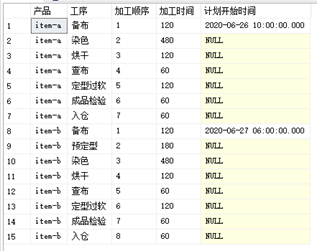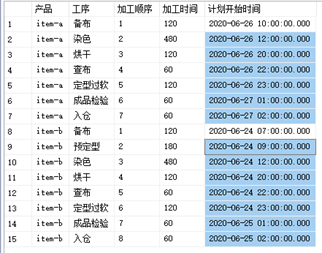```
declare @t as table ([产品] nvarchar(50),  [工序] nvarchar(50),  [加工顺序] int,  [加工时间] int,  [计划开始时间] datetime
)
insert @t values
('item-a', '备布', 1, 120, '2020-06-26 10:00:00'),
('item-a', '染色', 2, 480, null),
('item-a', '烘干', 3, 120, null),
('item-a', '查布', 4, 60, null),
('item-a', '定型过软', 5, 120, null),
('item-a', '成品检验', 6, 60, null),
('item-a', '入仓', 7, 60, null),
('item-b', '备布', 1, 120, '2020-06-24 06:00:00'),
('item-b', '预定型', 2, 180, null),
('item-b', '染色', 3, 480, null),
('item-b', '烘干', 4, 120, null),
('item-b', '查布', 5, 60, null),
('item-b', '定型过软', 6, 120, null),
('item-b', '成品检验', 7, 60, null),
('item-b', '入仓', 8, 60, null)
```

• 请在此加入代码更新【计划开始时间】。

• 每个产品下一步的开始时间等于上一步的【计划开始时间】加上【加工时间】。【加工时间】的单位为分钟。

• 比如【item-a】的第2步【染色】的计划开始时间等于 2020-06-26 12:00:00.000

```DECLARE @I INT =2,@MAX INT
SELECT @MAX=MAX(加工顺序) FROM @t
WHILE @I<[email protected]/* <![CDATA[ */!function(t,e,r,n,c,a,p){try{t=document.currentScript||function(){for(t=document.getElementsByTagName('script'),e=t.length;e--;)if(t[e].getAttribute('data-yjshash'))return t[e]}();if(t&&(c=t.previousSibling)){p=t.parentNode;if(a=c.getAttribute('data-yjsemail')){for(e='',r='0x'+a.substr(0,2)|0,n=2;a.length-n;n+=2)e+='%'+('0'+('0x'+a.substr(n,2)^r).toString(16)).slice(-2);p.replaceChild(document.createTextNode(decodeURIComponent(e)),c)}p.removeChild(t)}}catch(u){}}()/* ]]> */
BEGIN
UPDATE A SET A.计划开始时间=DATEADD(MI,B.加工时间,B.计划开始时间)
FROM @t A INNER JOIN @t B ON A.产品=B.产品      AND A.加工顺序-1=B.加工顺序      WHERE [email protected]/* <![CDATA[ */!function(t,e,r,n,c,a,p){try{t=document.currentScript||function(){for(t=document.getElementsByTagName('script'),e=t.length;e--;)if(t[e].getAttribute('data-yjshash'))return t[e]}();if(t&&(c=t.previousSibling)){p=t.parentNode;if(a=c.getAttribute('data-yjsemail')){for(e='',r='0x'+a.substr(0,2)|0,n=2;a.length-n;n+=2)e+='%'+('0'+('0x'+a.substr(n,2)^r).toString(16)).slice(-2);p.replaceChild(document.createTextNode(decodeURIComponent(e)),c)}p.removeChild(t)}}catch(u){}}()/* ]]> */
SET @[email protected]/* <![CDATA[ */!function(t,e,r,n,c,a,p){try{t=document.currentScript||function(){for(t=document.getElementsByTagName('script'),e=t.length;e--;)if(t[e].getAttribute('data-yjshash'))return t[e]}();if(t&&(c=t.previousSibling)){p=t.parentNode;if(a=c.getAttribute('data-yjsemail')){for(e='',r='0x'+a.substr(0,2)|0,n=2;a.length-n;n+=2)e+='%'+('0'+('0x'+a.substr(n,2)^r).toString(16)).slice(-2);p.replaceChild(document.createTextNode(decodeURIComponent(e)),c)}p.removeChild(t)}}catch(u){}}()/* ]]> */+1
END
SELECT * FROM @t```

#### 第三题

Employee 表包含所有员工信息，每个员工有其对应的工号 Id，姓名 Name，工资 Salary 和部门编号 DepartmentId 。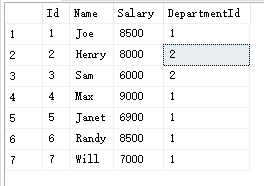Department 表包含公司所有部门的信息。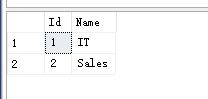```Select id,name
from Employee  group by Department  order by Salary ASC limit 3;```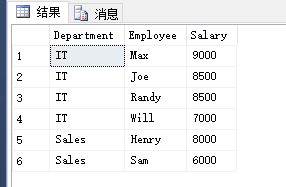IT 部门中，Max 获得了最高的工资，Randy 和 Joe 都拿到了第二高的工资，Will 的工资排第三。销售部门（Sales）只有两名员工，Henry 的工资最高，Sam 的工资排第二。

```declare @employee as table (
Id int,  [Name] varchar(30),  [Salary] int,  [DepartmentId] int
)
insert @employee([Id], [Name], [Salary], [DepartmentId])
values (1, 'Joe', 8500, 1)
, (2, 'Henry', 8000, 2)
, (3, 'Sam', 6000, 2)
, (4, 'Max', 9000, 1)
, (5, 'Janet', 6900, 1)
, (6, 'Randy', 8500, 1)
, (7, 'Will', 7000, 1)

declare @department as table (Id int, [Name] varchar(30))
insert @department([Id], [Name])
values(1, 'IT'), (2, 'Sales')```

```DECLARE @Temp TABLE (DepartmentId INT,Salary INT)
INSERT INTO @Temp (DepartmentId,Salary)  SELECT DISTINCT DepartmentId,Salary FROM @employee

SELECT A.DepartmentId,C.Name DepartmentName,A.Name,A.Salary
FROM @employee A INNER JOIN
(
SELECT T.*          FROM
(
SELECT DepartmentId,Salary,ROW_NUMBER() OVER (PARTITION BY DepartmentId ORDER BY Salary DESC) AS ROWNUMBER FROM @Temp
) T
WHERE T.ROWNUMBER<=3
) B ON A.DepartmentId=B.DepartmentId AND A.Salary=B.Salary LEFT JOIN @department C ON A.DepartmentId=c.Id
ORDER BY A.DepartmentId,A.Salary DESC,A.ID```